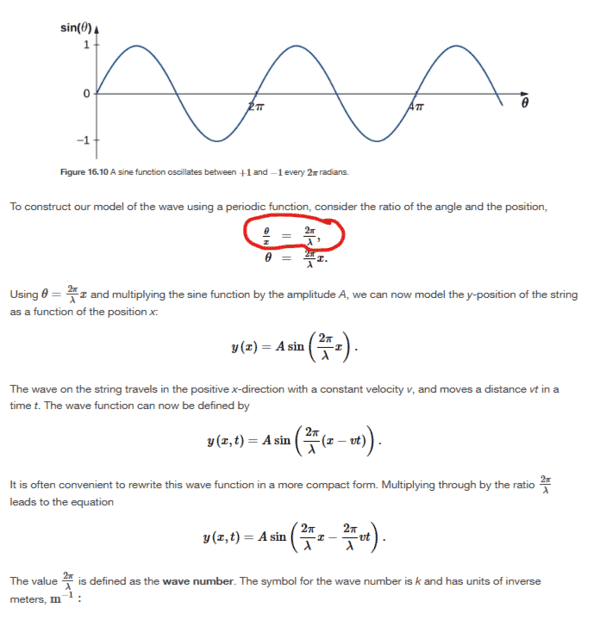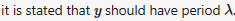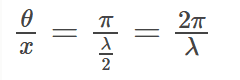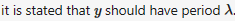# Deriving Wave Function for One-Dimensional Sinusoidal Wave

• ChiralSuperfields
In summary, the highlighted part of the graph does not seem to be derivable from the rest of the text.

#### ChiralSuperfields

Homework Statement
Please see below and https://openstax.org/books/university-physics-volume-1/pages/16-2-mathematics-of-waves for more details.
Relevant Equations
Where did they get the equation in circled in red from? It does not seem that it can be derived from the graph below.Many thanks

Last edited:
Figure 16.10 is just a graph of $\sin(theta)$. It is not referenced in the extract you have posted; why then you would expect the highlighted part to be directly derivable from a figure which is nowhere referred to? Perhaps look at the part of the text where the figure is actually referenced.

I suspect that, prior to the extract you have posted, it is stated that $y$ should have period $\lambda$. Sine, of course, has a period of $2\pi$, so to get a function with period $\lambda$ you have to use $\sin (2\pi x/\lambda)$, so that $\theta = 2\pi x/\lambda$ is equal to $2\pi$ when $x = \lambda$.

•Kumail Haider, topsquark, berkeman and 1 other person
pasmith said:
Figure 16.10 is just a graph of $\sin(theta)$. It is not referenced in the extract you have posted; why then you would expect the highlighted part to be directly derivable from a figure which is nowhere referred to? Perhaps look at the part of the text where the figure is actually referenced.

I suspect that, prior to the extract you have posted, it is stated that $y$ should have period $\lambda$. Sine, of course, has a period of $2\pi$, so to get a function with period $\lambda$ you have to use $\sin (2\pi x/\lambda)$, so that $\theta = 2\pi x/\lambda$ is equal to $2\pi$ when $x = \lambda$.
Thanks for your reply @pasmith! You meant to say that "it is stated that x should have a period of λ" instead of "" correct?

I guess they took an arbitrary point along the wave to for ratio of phase to wavelength which they also could of picked a point on the wave which has a π phase which has a wavelength λ/2.Many thanks

Callumnc1 said:
You meant to say that "it is stated that x should have a period of λ" instead of "" correct?
incorrect. In ##y=\sin(2\pi x/\lambda)##, it is the value of y that repeats as x increases by λ, so we say y has period λ.

•berkeman and ChiralSuperfields
haruspex said:
incorrect. In ##y=\sin(2\pi x/\lambda)##, it is the value of y that repeats as x increases by λ, so we say y has period λ.
Thank you @haruspex !